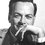# Proof without calculus: slope of parabolaThis is an image :D

For anyone who has learnt Calculus must know that the slope at any point on a parabola, for instance $y = \frac{1}{2} x^2$, is always equivalent to $\frac{dy}{dx} = x$. Yet I was just wondering if we can prove it without Calculus? Maybe with only geometry, vector, I don't know. If you would like to try this proof, I totally appreciate your effort, since I can't complete it on my own. Share your ideas here and let me know your wonderful thoughts, thanks! :D

For anyone who is curious to take a peek at this animation I made in Desmos(regarding this problem), feel free to check it out on this link: https://www.desmos.com/calculator/qw71a3cyylNote by Horace Hung
1 month ago

This discussion board is a place to discuss our Daily Challenges and the math and science related to those challenges. Explanations are more than just a solution — they should explain the steps and thinking strategies that you used to obtain the solution. Comments should further the discussion of math and science.

When posting on Brilliant:

• Use the emojis to react to an explanation, whether you're congratulating a job well done , or just really confused .
• Ask specific questions about the challenge or the steps in somebody's explanation. Well-posed questions can add a lot to the discussion, but posting "I don't understand!" doesn't help anyone.
• Try to contribute something new to the discussion, whether it is an extension, generalization or other idea related to the challenge.
• Stay on topic — we're all here to learn more about math and science, not to hear about your favorite get-rich-quick scheme or current world events.

MarkdownAppears as
*italics* or _italics_ italics
**bold** or __bold__ bold
- bulleted- list
• bulleted
• list
1. numbered2. list
1. numbered
2. list
Note: you must add a full line of space before and after lists for them to show up correctly
paragraph 1paragraph 2

paragraph 1

paragraph 2

[example link](https://brilliant.org)example link
> This is a quote
This is a quote
    # I indented these lines
# 4 spaces, and now they show
# up as a code block.

print "hello world"
# I indented these lines
# 4 spaces, and now they show
# up as a code block.

print "hello world"
MathAppears as
Remember to wrap math in $$ ... $$ or $ ... $ to ensure proper formatting.
2 \times 3 $2 \times 3$
2^{34} $2^{34}$
a_{i-1} $a_{i-1}$
\frac{2}{3} $\frac{2}{3}$
\sqrt{2} $\sqrt{2}$
\sum_{i=1}^3 $\sum_{i=1}^3$
\sin \theta $\sin \theta$
\boxed{123} $\boxed{123}$

## Comments

Sort by:

Top Newest

The slope is equal to rise over run $\Big( \frac{\Delta y}{\Delta x } \Big)$. Consider two points separated by a very small $x$ distance $\epsilon$.

$(x_1, y_1) = \big(x_0, \frac{1}{2} x_0^2 \Big) \\ (x_2, y_2) = \big(x_0 + \epsilon, \frac{1}{2} (x_0 + \epsilon)^2 \Big)$

The slope is the change in $y$ over the change in $x$.

$\text{slope} = \frac{y_2 - y_1}{x_2 - x_1} = \frac{\frac{1}{2} (x_0 + \epsilon)^2 - \frac{1}{2} x_0^2 }{(x_0 + \epsilon) - x_0}$

Expanding gives:

$\text{slope} = \frac{\frac{1}{2} x_0^2 + x_0 \epsilon + \frac{1}{2} \epsilon^2 - \frac{1}{2} x_0^2 }{\epsilon}$

Suppose $\epsilon$ is much smaller than $x_0$, meaning that the $\epsilon^2$ term in the numerator can be neglected. The expression then reduces to:

$\text{slope} = x_0$

- 1 month ago

Log in to reply

That looks suspiciously like a derivative to me! :D

- 1 month ago

Log in to reply

Isn't the very concept of "slope" the same thing as a derivative?

- 1 month ago

Log in to reply

True. :)

I think that's what the original question is referring to though: is there a way to define/calculate slope in, say, a purely geometrical way? @Avinash Rathore seems to have described something like that.

- 1 month ago

Log in to reply

Finally someone understands my question!!! @Steven Chase solution is in fact first principle in disguise, which I'd regard as part of Calculus :( Still a nice try. @Avinash Rathore solution is really similar to my original geometric proof, congrats!

Frankly I'd like to post my merely geometric proof here (without the slightest use of Calculus / Algebra / point-line distance formula), but the comment box is too small so it lefts as an exercise to readers

P.S. Just kidding, I'll try to post it somehow (Hint: @Avinash Rathore solution but with purely congruent triangles/locus of parabola/concepts of focus + directrix etc.)

- 1 month ago

Log in to reply

Awesome! :D

- 1 month ago

Log in to reply

The tangent and the normal at a point are the two angle bisectors of the lines (i) joining the focus and the point, and (ii) a line parallel to the y-axis passing through the point. Using the formula for the angle bisectors of a couple of lines,

          (Ax + By + C)^2/ (A^2 + B^2) = (ax + by + c)^2/ (a^2 + b^2)


(whose 2 roots give the tangent and normal), the line with the negative y intercept is the tangent. Simplifying, we can easily get the slope of the tangent as m = x.

NOTE - The above formula can be easily derived by equating the distance of any point on an angle bisector from the 2 given lines. This derivation does not require calculus.

Also, we note the above fact of the tangent and normal being angle bisectors by remembering the parabolic property that all lines parallel to the axis of the parabola get reflected or focused on to the focus which makes the normal at the point an angle bisector (and the tangent, being perpendicular to the normal is the other angle bisector).

Log in to reply

Appreciate your insight !

- 1 month ago

Log in to reply

×

Problem Loading...

Note Loading...

Set Loading...